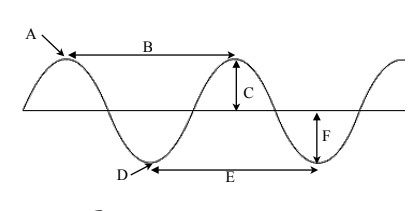7.1 Waves Worksheet
starstarstarstarstarstarstarstarstarstar
by Joseph Austin
| 31 Questions
Note from the author:
Waves
Multiple Choice. Some questions may have more than one answer. Select all that are correct.
1
1
(Select all that apply) Energy can be transferred
by the movement of materials
by the movement of heated gas
through matter by mechanical waves
through space by electromagnetic waves
2
1
What is a medium?
3
1
How is the amplitude of a wave related to the energy the wave carries?
4
1
Describe the difference between a compressional wave with a large amplitude and one with a small amplitude.
Diagram A. Refer to the picture and answer the series of questions.5
1
What shape of wave is shown in the figure?
6
1
Using the picture of the wave, which show(s) the WAVELENGTH?
A
B
C
D
E
F
7
1
Using the picture of the wave, which show(s) the AMPLITUDE?
A
B
C
D
E
F
8
1
Using the picture of the wave, which show(s) the CREST?
A
B
C
D
E
F
9
1
Using the picture of the wave, which show(s) the TROUGH?
A
B
C
D
E
F
Diagram B. Refer to the picture and answer the series of questions.10
1
Using the picture of the wave, which show(s) the WAVELENGTH?
A
B
C
11
1
Using the picture of the wave, which show(s) the COMPRESSION?
A
B
C
12
1
Using the picture of the wave, which show(s) the RAREFACTION?
A
B
C
13
1
1) An FM radio station broadcasts radio waves with a frequency of 100,000,000 Hz. What is the wavelength of these readio waves if they travel at a speed of 300,000,000 m/s? *submit answer in next question.
14
1
1) An FM radio station broadcasts radio waves with a frequency of 100,000,000 Hz. What is the wavelength of these readio waves if they travel at a speed of 300,000,000 m/s? *show work in previous question.
A
B
C
D
15
1
2) What is the frequency of a wave with a wavelength of 102 m, traveling at a speed of 51 m/s? *submit answer in next question.
16
1
2) What is the frequency of a wave with a wavelength of 102 m, traveling at a speed of 51 m/s? *show work in previous question.
0.5 Hz
2 Hz
51 Hz
5,202 Hz
17
1
3) A tuning form has a frequency of 280 hertz, and the wavelength of the sound produced in 1.5 meters. Calculate the velocity of the wave. *submit answer in next question.
18
1
3) A tuning form has a frequency of 280 hertz, and the wavelength of the sound produced in 1.5 meters. Calculate the velocity of the wave. *show work in previous question.
0.005 m/s
187 m/s
281.5 m/s
420 m/s
19
1
4) A wave is moving toward the shore with a velocity of 5.0 m/s. If its frequency is 2.5 Hz, what is its wavelength? *submit answer in next question.
20
1
4) A wave is moving toward the shore with a velocity of 5.0 m/s. If its frequency is 2.5 Hz, what is its wavelength? *show work in previous question.
0.5 m/s
2 m/s
12.5 m/s
17.5 m/s
21
1
5) The wake of a boat is moving at the velocity of 3 m/s. The waves are 1.5 meters apart. What is the frequency of the wave? *submit answer in next question.
22
1
5) The wake of a boat is moving at the velocity of 4 m/s. The waves are 1.5 meters apart. What is the frequency of the wave? *show work in previous question.
0.4 m/s
2.7 m/s
6 m/s
7.5 m/s
Measure. Use the grid of the graph to measure each attribute of the wave shown. Do not include units in your answer.23
1
How many waves are in the picture?
24
1
What is the amplitude of the wave?
25
1
What is the wavelength of one wave?26
1
How many waves are in the picture?
27
1
What is the amplitude of the wave?
28
1
What is the wavelength of one wave?29
1
How many waves are in the picture?
30
1
What is the amplitude of the wave?
31
1
What is the wavelength of one wave?